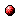# Optimistic optimization for continuous nonconvex piecewise affine functions

Reference:
J. Xu, T. van den Boom, and B. De Schutter, "Optimistic optimization for continuous nonconvex piecewise affine functions," Automatica, Mar. 2021. Article 109476.

Abstract:
This paper considers the global optimization problem of a continuous nonconvex piecewise affine (PWA) function over a polytope. This type of optimization problem often arises in the context of control of continuous PWA systems. In literature, it has been shown that the given problem can be formulated as a mixed integer linear programming problem, the worst-case complexity of which grows exponentially with the number of polyhedral subregions in the domain of the PWA function. In this paper, we propose a solution approach that is more efficient for continuous PWA functions with a large number of polyhedral subregions. The proposed approach is founded on optimistic optimization, which is based on hierarchical partitioning of the feasible set and which focuses on the most promising region when searching for the global optimum. The advantage of optimistic optimization is that one can guarantee bounds on the suboptimality with respect to the global optimum given a finite computational budget (e.g. the number of iterations). In particular, the gap between the best value returned by the proposed algorithm and the real optimum can be made arbitrarily small as the computational budget increases. We derive the analytic expressions for the core parameters required by optimistic optimization for continuous PWA functions. The efficiency of the resulting algorithm is illustrated with numerical examples.

Downloads:Online version of the paper

Bibtex entry:

@article{Xuvan:15-030,
author={J. Xu and T. van den Boom and B. {D}e Schutter},
title={Optimistic optimization for continuous nonconvex piecewise affine functions},
journal={Automatica},
month=mar,
year={2021},
note={Article 109476},
doi={10.1016/j.automatica.2020.109476}
}

Go to the publications overview page.

This page is maintained by Bart De Schutter. Last update: February 10, 2021.